# Future Learn MOOC - Activity and Confidence Analysis¶

### (Default Analysis Notebook)¶

From data on people that participated in a MOOC (Massive Open Online Course) we attempt to retrieve insights. The objective is to both: analyze the data from a run of such a MOOC, and also build a reasonably reusable tool to perform further analysis on similar data. We attempt to build a reasonably general data analysis tool that will allow a user to edit a few calls to output graphs with new relations. Also, we attempt to make the code robust enough so it does not rust too quickly with new data and new library updates.

A conservative approach as to what libraries to use will allow for a longer life of the code. We only use full version libraries, which should hence be always backward compatible with the same major versions. In our case any version of numpy 1, pandas 1 and matplotlib 3 shall run the code without issues. We also avoid the seaborn library in order to keep the code running for as long as possible, the seaborn library has not yet fully defined its interfaces.

In :
import numpy as np
import pandas as pd
import matplotlib
import matplotlib.pyplot as plt
%matplotlib inline
np.__version__, pd.__version__, matplotlib.__version__

Out:
('1.18.1', '1.0.1', '3.1.3')

Further we divide the work in three distinct parts. The jupyter notebook in which the work is contained follow the rule of placing dependencies above the dependent code, hence - even if the notebook appear to be run - one needs to run the cells above the current cell in order for the code to work. Remember that all code state is forgotten once you shutdown jupyter notebook/lab, in which case the notebook needs to be re-run. The parts of the notebook follow:

• The Data Model which consist of packaging the data we have into a consistent set of tables. For any compatible dataset this part will need to be reworked. Yet, once the dataset fits into the data model further analysis parts should just work.

• General Analysis build the common, and rather general, graphs about the participants of the MOOC. We build a few graphic tools along the way, tools that will be used in the following part.

• Extra Analysis is the part in which one can use the tools provided in the previous part to build his own graphs from the given data. The data processing in the Data Model part allows for extra columns to be passed all the way through the processing chain, this allows to build new graphs by just adding data.mooc-confidence-fl-windmill.svg

## Part 1: Data Model¶

In order to make the code as reusable as possible we establish a data model to follow. The operations on data will build a few common names which then will never be changed throughout the remaining of the code. Such discipline will allow us to reuse all analysis and graphics operations on a new set of data, provided we can fit the new set into the same data model.

The model itself will consist on a few tables:

• A fact table called response, with one main foreign key called participant_id, it represents the activity and responses of participants to the activity steps.

• A second fact table called step will follow the same model, it will have the main foreign key as participant_id as well.

• The main dimension will be called participant and use participant_id as its primary key. The participant dimension will aggregate unto itself everything we know about each participant from all source of data.

Both response and step facts will contain a time dimension upon themselves called week, a numeric and ascending value to filter against. In the response fact the primary key will be the uniqueness of participant_id, week, step and question. And in the step fact the primary key will be participant_id, week and step.

Albeit the two fact tables seem redundant to a good extent, the reason for the separation is that several participants may attend the MOOC but not answer the questions. The response fact accounts for more granular information on diligent participants, whilst the step fact accounts for all participants, including participants who only clicked on a few steps and gave up.

There is nothing holding the uniqueness of the keys described since we use pandas and not a SQL database. The onus on verifying the uniqueness is on the user. We add a few sanity checks after the data processing for this purpose, and these shall catch a good deal of issues if such appear.

### Current Data¶

In order to fit our data model we build the fact and dimension as follows:

• participant is built from enrolments data joined with the archetype-survey. the primary key learner_id is renamed to participant_id.

• response is constructed from the question-response data and joined with step-activity and aggregated comments. It's foreign key learner_id is renamed to participant_id.

• a third fact table comment is built from comments data and linked to the participant dimension. The author_id foreign key is renamed to participant_id for the linking purpose. This extra table is unlikely to appear in a different data set hence we leave it out of the data model. There is also no uniqueness (or primary key) for this data.

Note that all joins will be left joins from the participant dimension onto the fact tables, thus avoiding missing data on the dimension side.

The names of the CSV files may differ, one must remember to verify the file names, which must live in the same directory as the notebook. Also note that the filenames have the name of the MOOC course removed. The parse_dates= parameters are used for date and time columns, and allow us to work with times in an easier fashion. Whilst the index_col= takes the column with unique values, sometimes there is no column with unique values and sometimes the unique values are just meaningless ID numbers.

In :
archetype = 'archetype-survey-responses.csv'
country = 'countries.csv'
enrol = 'enrolments.csv'
question = 'question-response.csv'
activity = 'step-activity.csv'
video = 'video-stats.csv'
enrol = pd.read_csv(enrol, index_col='learner_id', parse_dates=['enrolled_at', 'fully_participated_at'])


### Sanity Checks¶

A few assumptions are made about the data before we process it. Some of these sanity checks will be repeated once we transition into the data model specified above but the earlier we find possible issues the better. Each of the tests below should not fail and should return True. If that is not the case then one must go back to the files and rectify.

There is only one type of question, namely multiple choice.

In :
len(question.question_type.unique()) ==  1

Out:
True

IDs for participants are unique.

In :
len(enrol.index.unique()) == len(enrol)

Out:
True

Indexes such as weeks and steps must be integers. Note that this also means that there are no null values as numpy does not allow for null integers.

In :
pd.api.types.is_integer_dtype(question.week_number)

Out:
True
In :
pd.api.types.is_integer_dtype(question.step_number)

Out:
True
In :
pd.api.types.is_integer_dtype(question.question_number)

Out:
True
In :
pd.api.types.is_integer_dtype(activity.week_number)

Out:
True
In :
pd.api.types.is_integer_dtype(activity.step_number)

Out:
True

### Transform (into the Data Model)¶

Now we can place the data we have into the data model we defined. The naming convention is that we will never use the same variable name for the loaded data and the data model table, this naming convention ensures that code cells can be re-run without too much care for the order in which they are re-run.

First we build the comment extra fact table. The table is not part of the data model but its contents will prove useful to enrich tables from the data model itself. We do not do many changes to the comment table from the CSV file.

In :
comment = remark.copy()
comment.drop('step', axis=1, inplace=True)
comment = comment.rename(columns={
'author_id': 'participant_id',
'week_number': 'week',
'step_number': 'step',
})
comment['text_length'] = comment.text.str.len()
cols = [i for i in comment.columns if i != 'text']  # prevent personal info leak
comment[cols][:3]

Out:
participant_id parent_id week step timestamp likes first_reported_at first_reported_reason moderation_state moderated text_length
id
59104690 0022e782-f726-4682-9a91-adb8401df949 NaN 1 1 2021-05-03 06:19:13+00:00 1 NaN NaN NaN NaN 300
59107936 ee7d449b-e4c5-44af-a890-61680d001828 NaN 1 1 2021-05-03 08:58:58+00:00 2 NaN NaN NaN NaN 446
59110647 d8f579a6-d8a2-4842-a4ea-cf6ecfa2d101 59104690.0 1 1 2021-05-03 10:45:09+00:00 1 NaN NaN NaN NaN 136

And we can now build the participant dimension, while at the same time enrich it with data from the archetype survey and the step comments.

The participant dimension defines all information we have about the participants, if more data about the participant themselves becomes available we can further extend this table with more columns.

In :
arch = archetype.reset_index().sort_values(by='responded_at', ascending=False).groupby('learner_id').first()
participant = enrol.join(arch)
participant = participant.rename_axis('participant_id')
participant = participant.join(tmp)
tmp = comment.rename(columns={'likes': 'total_likes'}).groupby(['participant_id']).sum()['total_likes']
participant = participant.join(tmp)
participant['total_likes'].fillna(0, inplace=True)
participant[:3]

Out:
enrolled_at unenrolled_at role fully_participated_at purchased_statement_at gender country age_range highest_education_level employment_status employment_area detected_country unlimited id responded_at archetype total_comments total_likes
participant_id
47c2add2-a127-40db-ae0e-12d2725a1a9a 2021-04-08 01:58:43+00:00 NaN NaN NaT NaN Unknown Unknown Unknown Unknown Unknown Unknown VN t 16725888.0 2021-04-08 02:04:11+00:00 Flourishers 0.0 0.0
09fcca1f-ff80-4c38-a81d-33efa02dd132 2021-05-01 09:11:12+00:00 NaN NaN 2021-05-25 15:58:18+00:00 NaN Unknown Unknown Unknown Unknown Unknown Unknown DE f NaN NaT NaN 1.0 1.0
6ba452a2-dc85-4891-ab10-8e4fe1fbe1e9 2021-05-05 11:55:31+00:00 NaN NaN NaT NaN female AT 18-25 secondary looking_for_work Unknown AT t NaN NaT NaN 0.0 0.0

With the step fact table we need to be careful to keep the uniqueness constraint we defined. One may suspect that the data could contain several completion times for each participant. In order to ensure the uniqueness we defined we always take only the first start and completion time given in the data.

Also, we explicitly add the time it took for a participant to complete the step. And whether there was a start and/or completion of the step at all. This data could be derived at any point later but for simplicity we keep it duplicated in the step fact table.

In :
step = activity.groupby(['learner_id', 'week_number', 'step_number']).first().copy()
step.reset_index(inplace=True)
step['completion_time'] = (step.last_completed_at - step.first_visited_at).dt.seconds
step['started'] = (~step.first_visited_at.isnull())
step['completed'] = (~step.last_completed_at.isnull())
step.drop('step', axis=1,  inplace=True)
step = step.rename(columns={
'learner_id': 'participant_id',
'week_number': 'week',
'step_number': 'step',
})
step[:3]

Out:
participant_id week step first_visited_at last_completed_at completion_time started completed
0 0022e782-f726-4682-9a91-adb8401df949 1 1 2021-05-03 06:07:33+00:00 2021-05-03 06:19:13+00:00 700.0 True True
1 0022e782-f726-4682-9a91-adb8401df949 1 2 2021-05-03 06:19:16+00:00 2021-05-03 16:12:25+00:00 35589.0 True True
2 0022e782-f726-4682-9a91-adb8401df949 1 3 2021-05-03 16:12:31+00:00 2021-05-03 16:20:29+00:00 478.0 True True

The response fact is the last one to add into the data model. Here the response column is quite messy, sometimes participants can answer several options, other times they can answer the same question several times. Since we assume that all answers will be numbers we take the mean of the participants' answers in every case.

On top of the answers to the questions themselves we enrich the response fact with the completion steps. Albeit it may be redundant to check whether a participant who has answered the questions has started and completed the same MOOC week, it may be useful to check the answers for participants who gave up and not completed the following MOOC week.

In :
response = question[['learner_id', 'week_number', 'step_number', 'question_number', 'response']].copy()
response.response = question.response.apply(lambda x: np.mean(list(map(int, x.split(',')))))
response = response.groupby(['learner_id', 'week_number', 'step_number', 'question_number']).mean()
response.reset_index(inplace=True)
response = response.rename(columns={
'learner_id': 'participant_id',
'week_number': 'week',
'step_number': 'step',
'question_number': 'question',
})
tmp = step[['participant_id', 'week', 'step', 'started', 'completed']]
response = response.merge(tmp, on=['participant_id', 'week', 'step'], how='left')
response[:3]

Out:
participant_id week step question response started completed
0 0022e782-f726-4682-9a91-adb8401df949 1 3 1 2.0 True True
1 0022e782-f726-4682-9a91-adb8401df949 1 3 2 4.0 True True
2 0022e782-f726-4682-9a91-adb8401df949 1 3 3 5.0 True True

The majority of the data is a time series, hence the majority of comparisons are best made by self joins at a different time, e.g. compare this week with next week. Self joins may be tricky to read in code, and it may therefore be quite difficult to change. There are several unneeded condition in the code that are placed in order to make the code robust against corner cases. If you are changing the code please leave theses conditions be no matter how obvious it seems that these are not needed for the data currently processed.

### Sanity Checks (on the data model)¶

We can perform a second set of sanity check on the data model itself. Again each of the tests below should not fail and should return True. If all goes according to plan (no failures) we expect the rest of the analysis to go smoothly.

In :
comment.text.isnull().sum() == 0

Out:
True

All participants are enrolled.

In :
participant.enrolled_at.isnull().sum() == 0

Out:
True

Participant identifiers are unique.

In :
len(participant.index) == len(participant.index.unique())

Out:
True

Everyone completed a step once or less (or got aggregated to one if completed a step several times).

In :
step.groupby(['participant_id', 'week', 'step']).size().sum() == len(step)

Out:
True

Every participant answered a question at most once.

In :
response.groupby(['participant_id', 'week', 'step', 'question']).size().sum() == len(response)

Out:
True

This completes the data modeling part. Let us say aloud the assumptions made about the data, assumptions that allow us to mathematically reason about the data.

• Questions of interest will always have numeric values, where the biggest value means better and the lowest means worse.

• A participant can only be enrolled once.

• The archetype questionnaire should only be answered once. If a participant answers the archetype questionnaire several times only the last set of answers is taken into account. (i.e. we force learner_id - before it is turned into participant_id - to be a PK for archetype)

• Questions of interest will always be about a participant's confidence in himself or some course of action. This allows us to make several further assumptions on response aggregation.

• Step question answers are only answered once. When several answers are performed by the same participant we take the mean of the answer. This is an assumption based on the fact that we are asking about a participant's confidence, and a change of heart is part of our evaluation.

And for the current dataset we also assume a few things that allows to fit the data into the data model.

• Country and Video data is not of any particular interest. Note that enrollment data already detects a country, which is a more reliable source.

## Part 2: General Analysis¶

A few general graphs about the course as a whole can be performed. Once we are done with those we start building a few tools to allow us analysis of the information about the participant's background. If the previous steps above have all succeeded, most code cells can be run at any time. Yet, the exception are the code cells at the end of this part where we define functions to be used for graphing. The most common error is to shutdown the notebook and then forget to re-run the code cell containing the graphing functions before attempting the graphs. Beware of that.

### Step Completion¶

How long it did take to complete a step? In other words how long did it take between first visiting a step and then clicking the "complete" button at the end.

Many things could happen between these two occurrences: one could go make a coffee and come back, one could forget about the course and never click the complete, or even one could return to the course several days later and only then click complete. To allow us to see information from the graphs of this data we use two tricks: first we use a logarithmic scale on the vertical axis (number of completed steps), and second we throw away any completions longer than $3$ hours (we assume that after three hours someone just to forgot about the step).

In :
fig, ax = plt.subplots(figsize=(16, 9))
three_hours = 60*60*3
completed_time = step.completion_time[step.completion_time < three_hours]
ax.hist(completed_time / 60, bins=64, color='firebrick');
ax.set_yscale('log')
ax.set(title='Total Step Completion Time', xlabel='minutes', ylabel='total completions');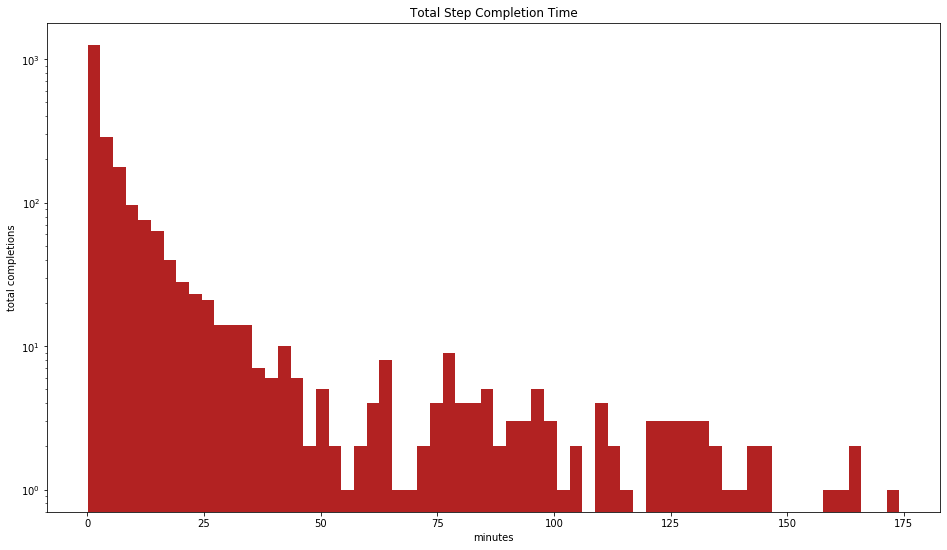Figure 1: Distribution of log step-time. We see the distribution of the time it took to complete any step of the course. All steps are bundled together and the vertical scale is logarithmic, which allows us to visualize both the small and large times. There is a hard cutoff at $180$ minutes (three hours), after which we consider the participant to have forgotten about the step.

Originally we have expected a Gaussian distribution for the completion times, with a well defined peak around the common completion times (a few minutes perhaps). Yet, we get a Poisson distribution suggesting either that most participants would rush through the steps or would complete the steps fast at first and only then come back to go through the step slowly.

The above was a generalization for all steps together. If we build such a graph for every single step separately we may be able to identify more interesting and less interesting steps based on the time spent on them.

In :
all_steps = dict([(k,v) for k,v in step.groupby(['week', 'step'])])
three_hours = 60*60*3
fig, ax = plt.subplots(int(np.ceil(len(all_steps)/4)), 4, sharex=True, sharey='row', figsize=(20, 16))
for i, s in enumerate(all_steps):
(weeknum, stepnum) = s
cur_step = all_steps[s]
this_step = cur_step.completion_time[cur_step.completion_time < three_hours]
ax.flat[i].hist(this_step / 60, bins=64, color='indianred')
ax.flat[i].set_ylabel(f'week {weeknum} step {stepnum}', rotation=45, ha='right')
ax.flat[i].set_yscale('log')
x1, x2, y1, y2 = ax.flat[i].axis()

# fix last row ticks (avoid a matplotlib bug with empty plots)
ymin, ymax = ax.flat[-1].get_ylim()
ax.flat[-1].set_ylim(max(ymin, 0.1), min(len(step), ymax))
fig.suptitle('Individual Step Completion Time', y=.9, fontsize=14)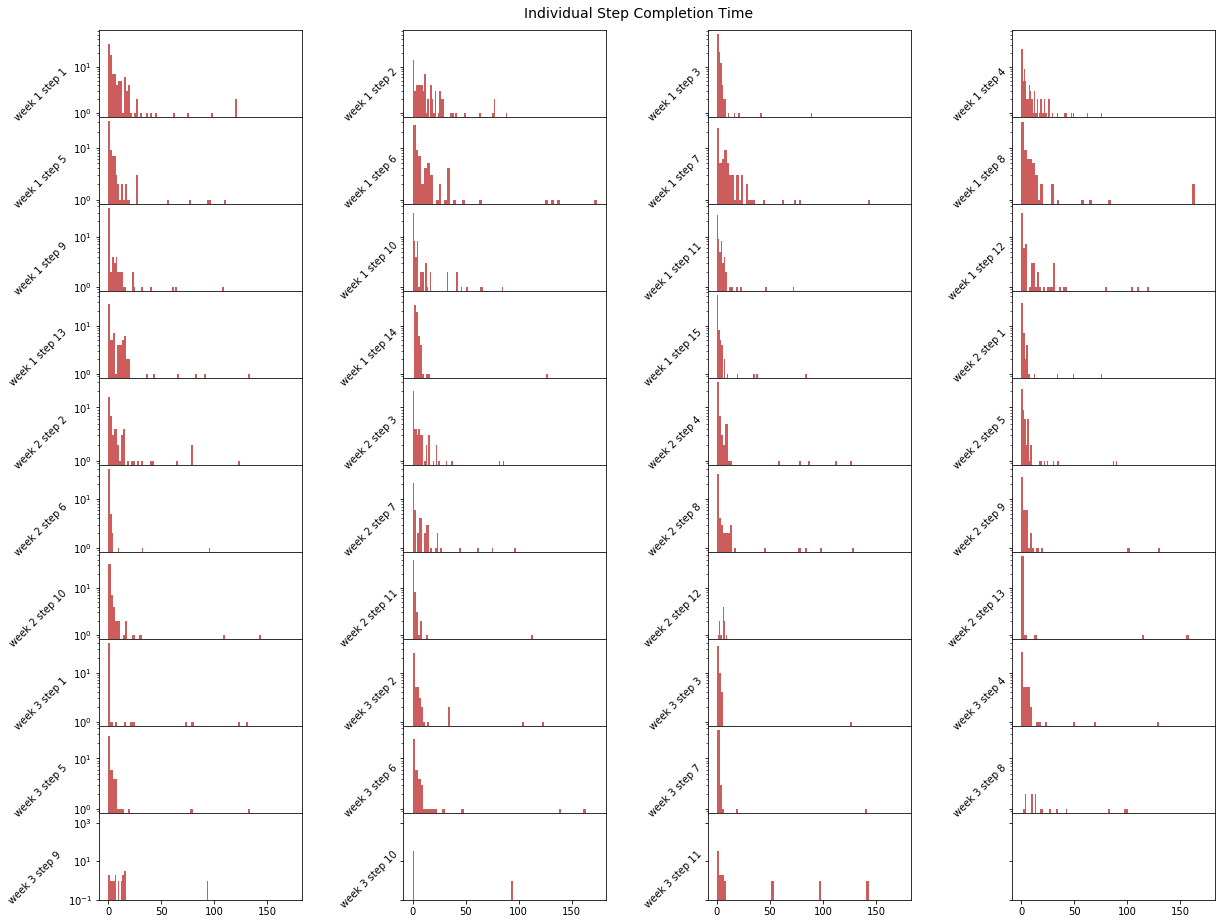Figure 2: Distribution of times per step. The same log step-time is plotted divided by the course steps. Each steps is given its own distribution of completion times by participants.

The most interesting steps should have a more spread distribution. One can argue that - statistically - the steps where people stopped a while to think indicate that something sparkled interest. Note that as the steps progress some participants will give up, hence the statistical value decreases as the steps progress. Also note that the last few rows of graphs are scaled lower than the rest, this is both to avoid statistical conclusions from comparing top graphs (in which many more people participated) with bottom graphs. On top of that this scaling of later rows allow us to extend the code to as many steps as possible in the data, whilst avoiding a known bug in matplotlib that would make the last row out of scale if an empty graph was present.

Nevertheless, to compare the people who gave up during the course and not participated in later steps we can attempt a different graph. Since most people give up between weeks of a course, we will plot the completion times by week and color differently the participants that followed the course to the next week.

In :
def plot_hist(step, week, ax):
cur_step = step[step.week == week]
next_step = step[step.week == week + 1][['participant_id', 'step']]
if len(next_step):  # hardened code, the condition is not needed but just in case
next_step = next_step.groupby('participant_id').count()
next_step.columns = ['stayed']
next_step['stayed'] = True
next_step = next_step.reset_index()
combined = pd.merge(cur_step, next_step, on='participant_id', how='left')
combined['stayed'].fillna(False, inplace=True)
else:
combined = cur_step.copy()
combined['stayed'] = False

ctime = combined.completion_time
three_hours = 60*60*3
ctime = ctime[ctime < three_hours]
_, bins, _ = ax.hist(ctime / 60, bins=64, color='coral', alpha=.7)

stayed = combined[combined.stayed]
if len(stayed):  # again not needed, but leave it hardened just in case
ctime = stayed.completion_time
ctime = ctime[ctime < three_hours]
_, bins, _ = ax.hist(ctime / 60, bins=bins, color='teal', alpha=.9)

ax.set_ylabel(f'week {week}')
ax.set_yscale('log')

n = step.week.max()
fig, ax = plt.subplots(n, 1, sharex=True, figsize=(20, 3*n))
for i in range(n):
plot_hist(step, i+1, ax.flat[i])

ax.flat[-1].set_xlabel('minutes')
ax.flat.set_title('Completion Time and Dropout')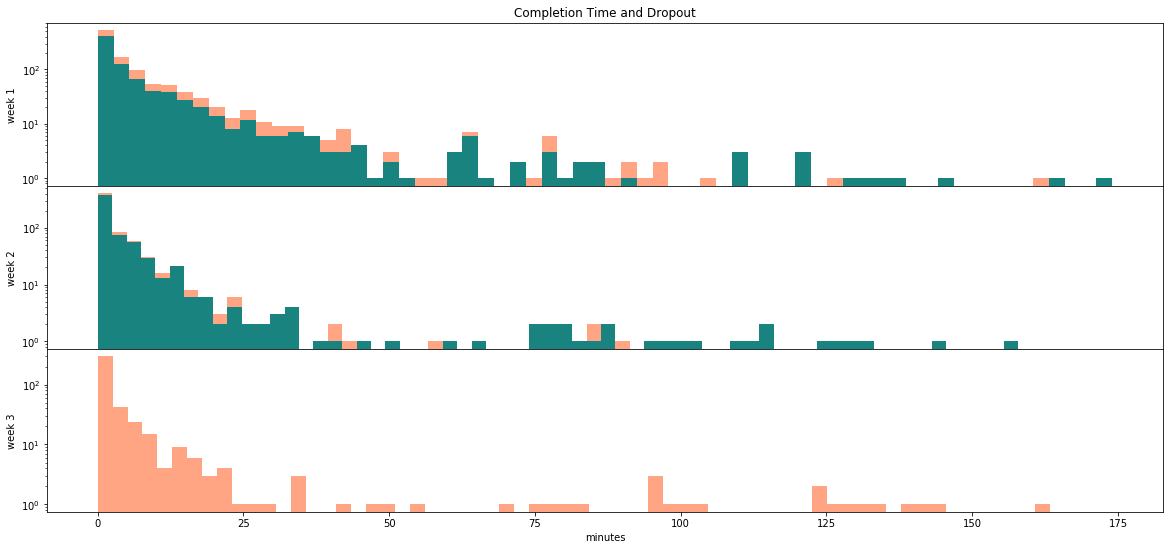Figure 3: Log step-time distribution by week. In orange we see the participants that gave up that week (in the last week all participants give up as it is the end of the MOOC). In green we see the participants that stayed for the following week. Note the vertical logarithmic scale, the higher on the graph a difference between orange and green is present the more participants gave up.

Each week (each graph) we see how many participants have completed the assigned steps within a certain number of minutes. Each week less and less participants complete the steps as participants give up the MOOC. Participants that spend little time on the step are more likely to give up and not follow the next week. The left hand side where the completion times are small decreases fast, whilst the right hand side remains reasonably constant.

From all three graphs, a viable hypothesis is that at the very beginning participants spend time on the steps out of curiosity, later the time spent is likely proportional to the engagement of a step/session. Notably, steps $3.7$, $3.8$ and $3.9$ appear to be quite engaging.

As for participants giving up, we can plot only that instead.

In :
this_step = step[['participant_id', 'week', 'step', 'started']]
next_step = this_step.copy()
next_step.step -= 1
advanced = pd.merge(this_step, next_step, on=['participant_id', 'week', 'step'], how='left')

fig, ax = plt.subplots(figsize=(20, 9))
ax.bar(range(len(bars)), bars.started.values, color='coral', width=1)
ax.set_xticks(range(len(bars)))
labels = [f'week {i} step {j}' for i, j in bars.index]
ax.set_xticklabels(labels, rotation=45, ha='right')
ax.set_ylabel('participants')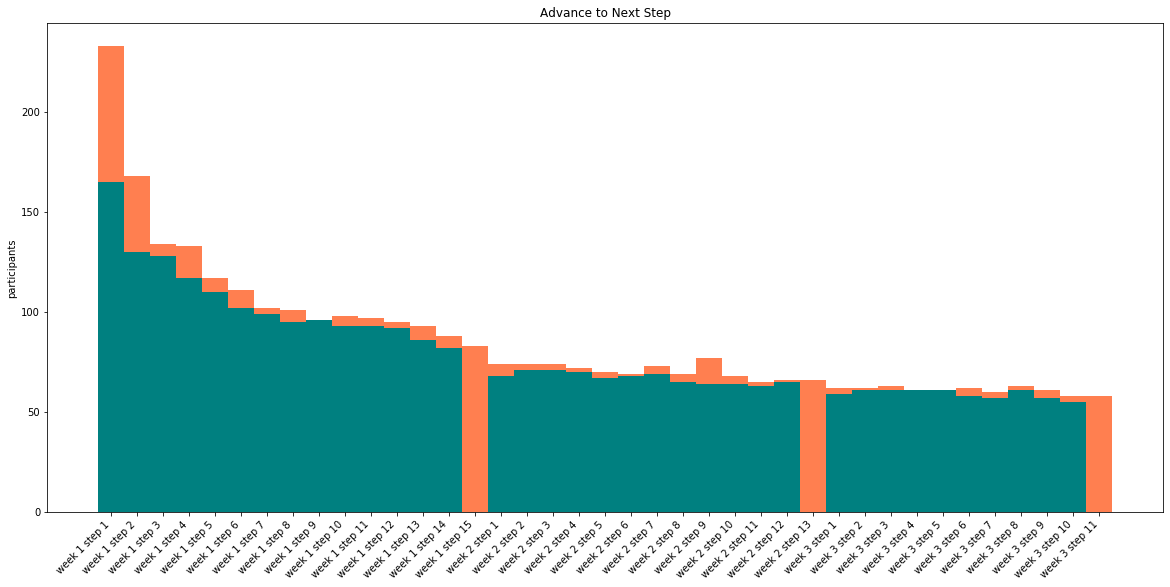Figure 4: Participant Advance to the Next Step. The orange bars report how many participants started a (current) step, whilst the green bars report how many participants started the current step and also started the next step. The difference between the green and orange bars is how many participants did not advance directly to the next step; remember that the orange bars are behind the green bars. In the last step of each week we do not plot a green bar, which allows us to see the week splits.

Most differences between orange and green bars will be participants that gave up on the course on the given step. Albeit the differences may result from different cases too, for example a participant that jumps over a step will be plotted as orange in the previous step whilst he/she will continue to be reported as green in the following step. In the current data we can see a large number of participants giving up early in the course but then the remainder of participants did keep up with the course.

### Participant Confidence Responses¶

Next we will look at the responses to the confidence questions present in the course questionnaires. In order to allow the notebook to be used for different courses one needs to explicitly state which week and step the questionnaires of interest are present.

One assumption we make is that question $1$ in one questionnaire is of similar spirit as question $1$ in the next questionnaire, and so on. In other words, we assume that we can compare the same question numbers across different questionnaires.

CONFIGURATION NEEDED. The following variable must be set to the groupings of week and step in which the questionnaires of our interest are. It must be a list of tuples (or equivalent) in the format (week_number, step_number). For example, confidence = [(1, 3), (2, 6)] tells us that the questionnaires of interest are in week 1 step 3 and week 2 step 6. There is no limitation as to how many questionnaires can be added or that there must be a questionnaire per week. As many questionnaires with any combination of week can be added. A chronological order is not needed but recommended as the graphs below assume chronological order.

In :
# this is week 1 step 3, week 2 step 6 and week 3 step 7
confidence = [(1, 3), (2, 6), (3, 7)]


We then build a data structure based on the configuration provided. Note that we do not fully sanity check the configuration, it may happen that the following works without error and the graphs below become empty. If that happens the most likely cause is the incorrect setting of the variable above.

The sanity check below shall capture most (but not all) such issues. The following shall not error and shall print the number of question responses per questionnaire. If any of the numbers is $0$ (zero) then either a misconfiguration happened and/or it points to an empty questionnaire. The check will not catch questionnaires with only some questions missing.

In :
all_steps = []
for w, s in confidence:
all_steps.append(response[(response.week == w) & (response.step == s)])
list(map(len, all_steps))

Out:
[416, 103, 175]

A questionnaire can be composed from several questions, next we plot the questionnaires against questions and build a histogram distribution of answers to each.

In :
bins = int(response.response.max())
n_steps = len(all_steps)
n_questions = max(map(lambda x: len(x.question.unique()), all_steps))
fig, axs = plt.subplots(n_steps, n_questions, sharex=True, sharey=True, figsize=(22, n_steps*3))

for i in range(n_steps):
for j in range(n_questions):
this_step = all_steps[i]
this_step = this_step[this_step.question == j + 1]
next_step = all_steps[i+1] if i+1 < len(all_steps) else None
ax = axs[i, j]
_, edges, _ = ax.hist(this_step.response, bins=bins, color='orchid')
if next_step is not None:
next_step = next_step[['participant_id', 'question']]
next_step = next_step[next_step.question == j + 1].copy()
next_step.rename(columns={'question': 'next_week_question'}, inplace=True)
qtmp = pd.merge(this_step, next_step, on='participant_id', how='inner')
ax.hist(qtmp.response, bins=bins, color='seagreen')
if j == 0:
ax.set_ylabel(f'questionnaire {i+1}\nnumber of participants')
if i == 0:
ax.set_title(f'question {j+1}')
if i+1 == n_steps:
middles = (edges[:-1] + edges[1:]) / 2
ticks = np.array(list(range(bins))) + 1
ax.set_xticks(middles)
ax.set_xticklabels(ticks)
ax.set_xlim(middles-1, middles[-1]+1)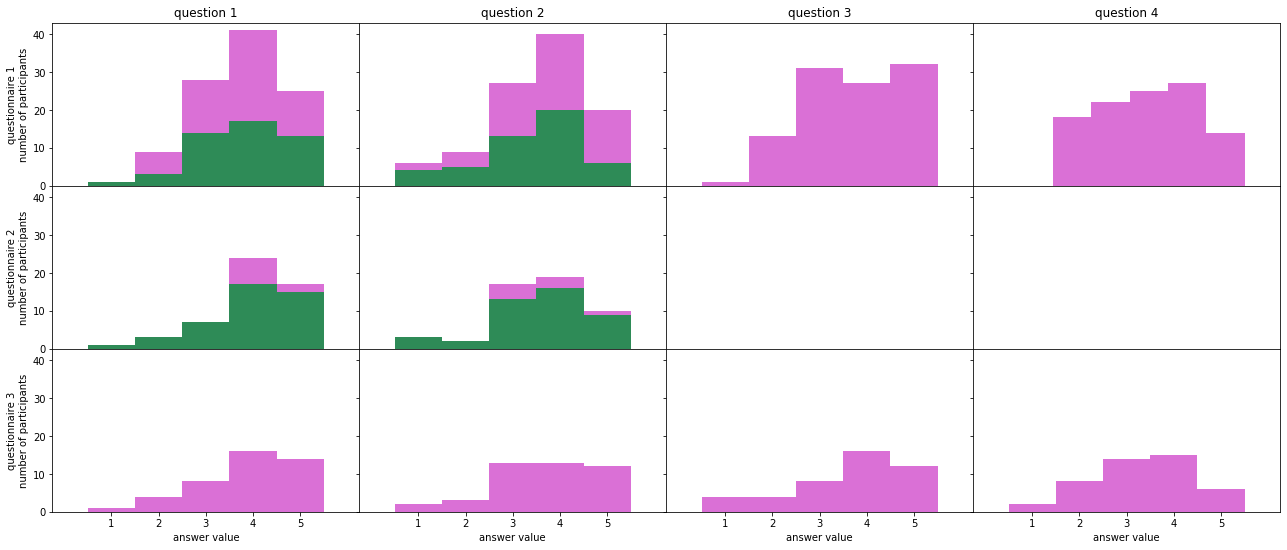Figure 5: Distributions of Answers Across Questionnaires. The higher the answer value the higher is the confidence of the participant in that area. The difference between colors again shows how many participants gave up on that week, in this case we take a participant that gave up by checking if he/she has answered the same question on the following questionnaire. The scales between all graphs are the same, the height can be compared to account for the change in confidence.

From the current data, a fair hypothesis is to say that the positive change in confidence happens at the end of the MOOC. Participants who did stay only for the beginning do not show a change in confidence. But the participants that stayed to the end show a shift to the right in several places between the graphs. Note that a change in confidence if the difference between a previous graph (above from current) in green to the next graph (current) in indigo.

### Participant Statistics¶

Until now all statistics have been generalized across all participants, yet we have information that allows us to divide the participants into groups. Groups by age, profession, education, whatnot; Then we can build temporal graphs for the questionnaires on each group.

In order to automate the graphing we will build a data structure for question answers similar to the one used in the previous graph, but this time we will also build a structure for the participants that did advance in the course. Advanced to the next questionnaire, and the ones who advanced through the complete course.

In :
# We could loop only once for everything,
# but that would assume that we always have only a single step of interest per week.
# Looping several times allow for less assumptions on the data.
all_resp = []
all_resp_comp = []
for w, s in confidence:
all_resp.append(response[(response.week == w) & (response.step == s)])


And since we are building the data structures we will construct an analogous structure for the started steps by the participants.

In :
all_step = []
for w in range(step.week.max()):
all_step.append(step[step.week == w+1])


Once the structures are built we will join the tables with the participant table which will allow us to filter by the statistics we may want.

Note that this is all dependent on the setting of confidence configuration a few steps above.

In :
def enrich_data(step_resp):
enriched = pd.merge(participant, step_resp, left_index=True, right_on='participant_id', how='right')
# sanity check
if len(step_resp) != len(enriched):
print('WARNING: incomplete RIGHT join')
return enriched


We have all the building blocks and we run them all together. Once again remember the order of the notebook, the code below is heavily dependent on the code cells above.

A few sanity checks are printed but these will not catch all possible issues, notably problems with the confidence variable of weeks and steps where the questionnaires are located. If something goes wrong here, the first place to check is the configuration a few steps back.

We build and enrich the responses structure.

In :
first_resp = all_resp
first_resp = first_resp[['participant_id', 'question', 'response']].copy()
first_resp.rename(columns={'response': 'first_response'}, inplace=True)
last_resp = all_resp[-1]
last_resp = last_resp[['participant_id', 'question', 'response']].copy()
last_resp.rename(columns={'response': 'last_response'}, inplace=True)
for i in range(len(all_resp)):
this_resp = all_resp[i]
next_resp = all_resp[i+1] if i+1 < len(all_resp) else None
if next_resp is not None:
# this grouping here is the main reason why we split into lists
next_resp = next_resp[['participant_id', 'question', 'response']].copy()
next_resp.rename(columns={'response': 'next_response'}, inplace=True)
adv_step = pd.merge(this_resp, next_resp, on=['participant_id', 'question'], how='inner')
else:
# last element will always be empty
df = this_resp.drop(this_resp.index)
df['next_response'] = np.nan
df['increase'] = .0
comp_resp = pd.merge(this_resp, first_resp, on=['participant_id', 'question'], how='inner')
comp_resp = pd.merge(comp_resp, last_resp, on=['participant_id', 'question'], how='inner')
comp_resp['total_increase'] = comp_resp.last_response - comp_resp.first_response
all_resp_comp.append(comp_resp)

all_resp = list(map(enrich_data, all_resp))
all_resp_comp = list(map(enrich_data, all_resp_comp))
# sanity check
print('We got', len(all_resp_adv), 'questionnaires of interest')
print('And we got', len(all_resp_comp), 'questionnaires with completions')

We got 3 questionnaires of interest
And we got 3 questionnaires with completions


And build the analogous code for the started steps.

In :
for i in range(len(all_step)):
this_step = all_step[i]
next_step = all_step[i+1] if i+1 < len(all_step) else None
if next_step is not None:
next_step = next_step[['participant_id', 'started']].copy()
next_step = next_step.groupby('participant_id').sum().reset_index()
next_step.rename(columns={'started': 'started_next'}, inplace=True)
else:
# last element will always be empty
df = this_step.drop(this_step.index)
df['started_next'] = False

all_step = list(map(enrich_data, all_step))
# sanity check
print('And we got', len(all_step_adv), 'weeks in the course')

And we got 3 weeks in the course


### Violin Graph¶

In order to display and compare distributions in time we will use groups of violin graphs. A violin graph displays two probability distributions on each side of a vertical divide, Each distribution follows a probability hence the area under each distribution is always the same, this means that once can compare the shape of the distribution but cannot compare the total height.

Several graphic libraries have violin graph engines but these engines can be unstable, at the time of writing all graph engines with extensive violin graph options are either in alpha or beta stages of development and can change their APIs without warning. To make the code as long lived as possible we write our own (tiny) violin graph engine on top of the basic violin graph functionality in the stable matplotlib library. The following code builds violin graphs.

In :
def half_violin(x, y, *args, ax=None, left=False, mean=None, meadian=None, color=None, alpha=None, **kwargs):
# force a clean plot so we can add these manualy
kwargs['showmeans'] = False
kwargs['showextrema'] = False
kwargs['showmedians'] = False
# allow for lists and single values
if type(alpha) is not list and type(alpha) is not tuple and type(alpha) is not np.ndarray:
alpha = [alpha]*len(x)
if type(color) is not list and type(color) is not tuple and type(color) is not np.ndarray:
color = [color]*len(x)
# filters out empty arrays (sanity check first)
filtered = zip(*[(x, y, c, a) for x, y, c, a in zip(x, y, color, alpha) if len(x) > 0])
if not list(filtered):  # everything is empty, bail
return
x, y, color, alpha = zip(*[(x, y, c, a) for x, y, c, a in zip(x, y, color, alpha) if len(x) > 0])
params = ax.violinplot(x, y, *args, **kwargs)
for i, b in enumerate(params['bodies']):
if not b.get_paths():  # empty graph - violinplots do not work well with missing data
continue
# get the center
m = np.mean(b.get_paths().vertices[:, 0])
clips = [m, np.inf]
if left:
clips = [-np.inf, m]
# modify the paths to not go further right than the center
b.get_paths().vertices[:, 0] = np.clip(b.get_paths().vertices[:, 0], *clips)
if color[i]:
b.set_color(color[i])
if alpha[i]:
b.set_alpha(alpha[i])

def double_violin(xl, xr, y, *args, ax=None, color_left=None, color_right=None, **kwargs):
half_violin(xl, y, *args, ax=ax, left=True, color=color_left, **kwargs)
half_violin(xr, y, *args, ax=ax, color=color_right, **kwargs)


The question versus questionnaire graph - similar to the previous one above - will allow us to understand how to read a group of violin graphs in time. We will call one side of the violin (a distribution) a half-violin and a double sided violin (two distributions) a double-violin. (Please ignore the mathematical inconsistency of these names.)

In order to see statistical significance across half-violins distributed from a different number of data points we add transparency based on the amount of data. The colors are more opaque (brighter in a white background) as more data is used. For the case of a MOOC, where people give up as the course progresses, we see more and more transparent violins as we go right (forward chronologically).

In :
n_questions = max(map(lambda x: len(x.question.unique()), all_resp))
fig, ax = plt.subplots(n_questions, 1, sharex=True, figsize=(20, 6*n_questions))

ticks = list(range(len(all_resp)))
labels = list(map(lambda x: f'questionnaire {x+1}', ticks))
for i in range(n_questions):
fil = lambda x: x[x.question == i+1].response.dropna().reset_index(drop=True)
left = list(map(fil, all_resp))
right = list(map(fil, all_resp_comp))
atmp = np.array(list(map(len, left)))
alpha = atmp / (atmp.sum() * 2) + .5  # weight size for alpha values
double_violin(left, right, ticks, ax=ax[i], color_left='khaki', color_right='orchid', alpha=alpha)
ax[i].set_ylabel(f'question {i+1}')

ax[-1].set_xticks(ticks)
ax[-1].set_xticklabels(labels, rotation=45, ha='right')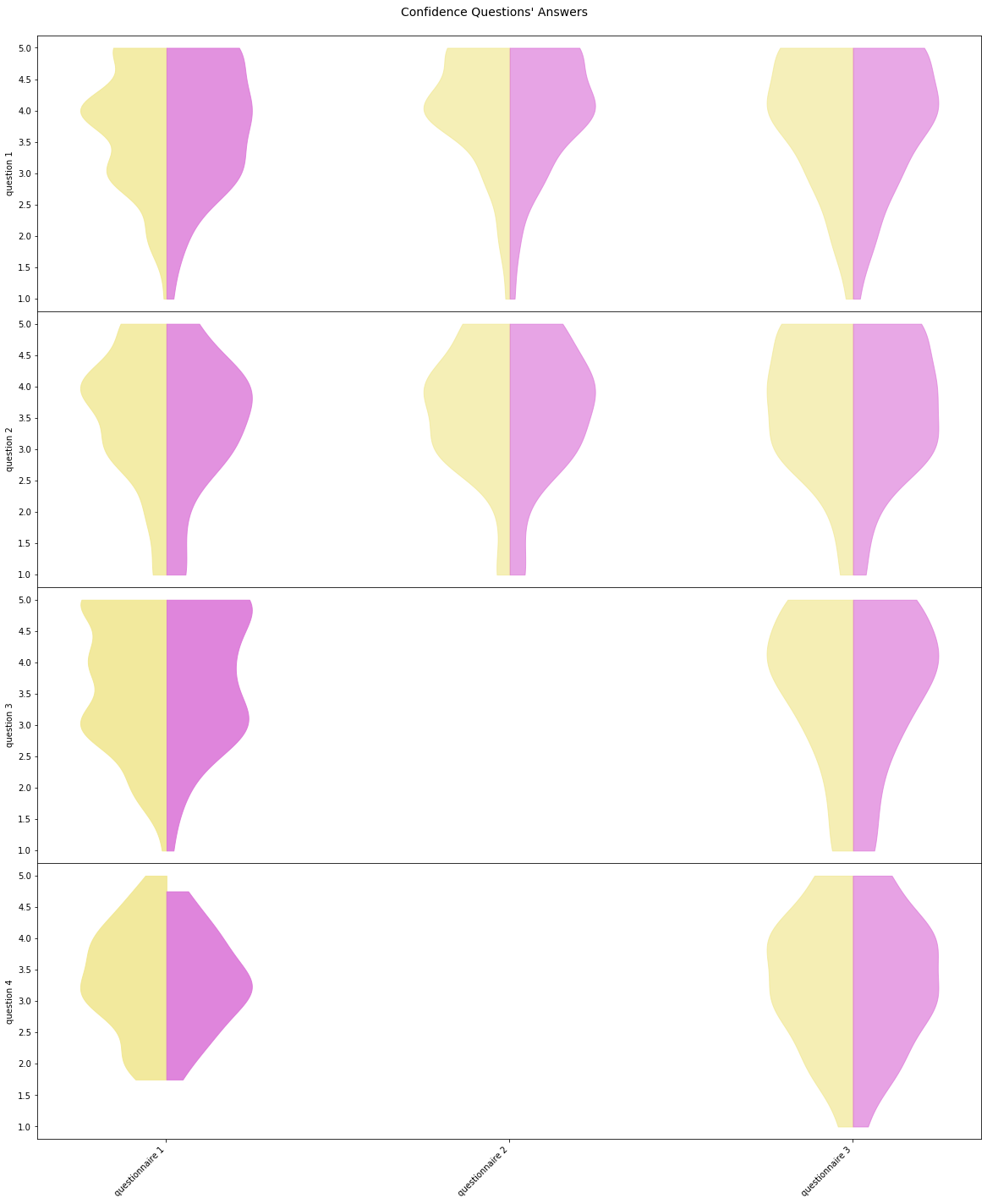Figure 6: Violin graphs for Questionnaire Answers to each Question. We see similar distributions as in the previous graphs but the violin graphs are probabilistic distributions as opposed to the previous raw histograms. Where before missing questions from questionnaires appeared as empty graphs here the missing questions appear as missing half-violins. Note that the last half-violin will always be a half as there is no next week/questionnaire to advance to.

From the current data we see the same general trend towards upper answers as we saw in the raw histograms. Remember that the violin graphs allow us to inspect the distribution shape with more precision than raw histograms but the height of the distribution is meaningless when compared because all distributions have the same area. The total amount of data points is shown by the brightness of the color, by the opaqueness, where less data makes for transparent (bleak) graphs.

### Generalized Statistics¶

The main reason for the violin graphs is that we can split the data across statistics of participants and plot a set of violins for each split. We can take any column from the participant table of the data model.

In :
participant.columns

Out:
Index(['enrolled_at', 'unenrolled_at', 'role', 'fully_participated_at',
'purchased_statement_at', 'gender', 'country', 'age_range',
'highest_education_level', 'employment_status', 'employment_area',
'detected_country', 'unlimited', 'id', 'responded_at', 'archetype',
dtype='object')

Let us take any of the participant statistics and check the values. We can do this with value_counts.

In :
participant['highest_education_level'].value_counts()

Out:
Unknown                 330
university_degree        28
university_masters       15
tertiary                  6
university_doctorate      4
secondary                 3
Name: highest_education_level, dtype: int64

Note that the list of values is always in decreasing order, which also means a decreasing statistical value. For each grouping in the statistic we can plot a set of violin plots as we did before for all questionnaires. We will do so in the decreasing order of statistical significance and the graphs shall vanish as we go from left to right.

The graphs may become unreadable if there are too many groupings hence we will make a default limit of a maximum of $13$ groupings. The value is arbitrary and woks well for a standard screen size (around $20$ inches wide) and $3$ questionnaires. One can use the limit= parameter to adjust the limit as needed. One can even say limit=None to remove the limit altogether and plot all groupings even if they exceed $13$ groupings.

In :
# to keep things readable, we limit by 13 biggest values by default
def plot_response(stat, dropouts=False, start=0, limit=13):
n_questions = max(map(lambda x: len(x.question.unique()), all_resp))
estat = participant[stat].value_counts()
fig, ax = plt.subplots(n_questions, 1, sharex=True, figsize=(20, 6*n_questions))
if dropouts:

colors = [('khaki', 'orchid'), ('seagreen', 'firebrick')] * int(np.ceil(len(estat) / 2))
ticks = list(range(len(all_resp)))
complete_ticks = []
labels = list(map(lambda x: f'questionnaire {x+1}', ticks))
complete_labels = []
walk = enumerate(estat.index)
if limit:
walk = list(walk)[start:limit]
for n_stat, cur_stat in walk:
max_respondents = np.array(*len(all_resp))
for i in range(n_questions):
fil = lambda x: x[(x.question == i+1) & (x[stat] == cur_stat)].response.reset_index(drop=True)
left = list(map(fil, all_resp))
# weight size for alpha values (prevent division by zero)
atmp = np.array(list(map(len, left)))
alpha = atmp / (atmp.sum() * 2 + .1) + .5
cleft, cright = colors[n_stat]
double_violin(left, right, ticks, ax=ax[i], color_left=cleft, color_right=cright, alpha=alpha)
ax[i].set_ylabel(f'question {i+1}')
max_respondents = list(map(lambda x: max(*x), zip(max_respondents, atmp)))
#print(max_respondents)
complete_ticks += ticks
ticks = list(map(lambda x: x+n_questions+1, ticks))
def mklabels(l):
q, stat = l
return f'{cur_stat} ({stat}/{estat[cur_stat]}) - ' + q
complete_labels += list(map(mklabels, zip(labels, max_respondents)))

ax[-1].set_xticks(complete_ticks);
ax[-1].set_xticklabels(complete_labels, rotation=45, ha='right')
fig.suptitle(stat, y=.9, fontsize=14)
return estat


We can now plot against whichever statistic we may wish with a simple function call.

In :
plot_response('highest_education_level')

Out:
Unknown                 330
university_degree        28
university_masters       15
tertiary                  6
university_doctorate      4
secondary                 3
Name: highest_education_level, dtype: int64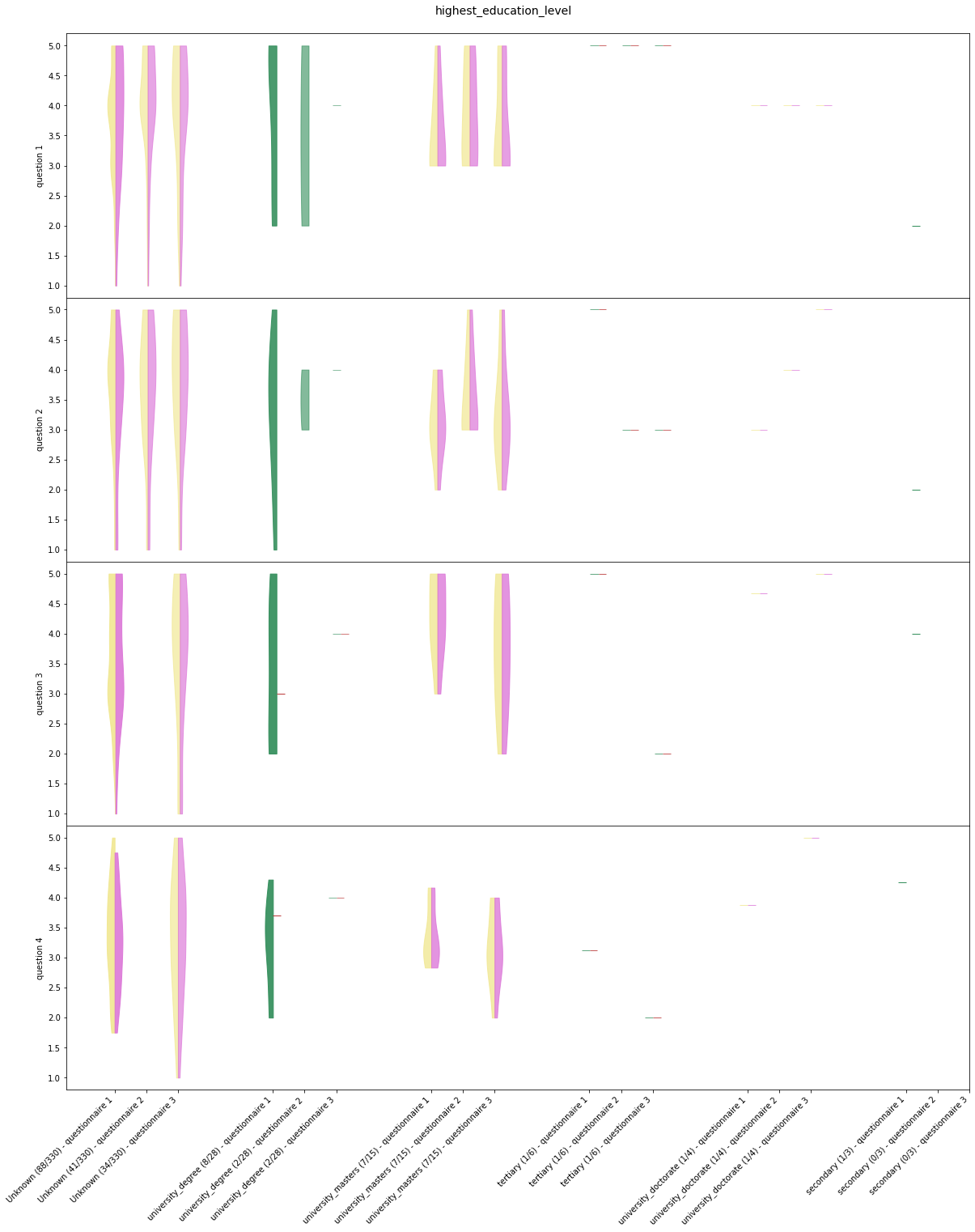Figure 7: Violin Graphs for each Highest Education Level Grouping. The graphs use less and less statistically valuable groupings as we read from left to right. The transparency of the graphs indicates the statistical significance within that grouping (amount of data used). While the significance across groupings can be estimated from the bottom labels. Each label contains the size of the complete grouping, hence it is the amount of data used for the complete set of violin graphs. Each violin graph set is separated by the color convention, the sets alternate between yellow (khaki) left / pink (orchid) right and green (sea green) left / orange (firebrick) right.

The function that generates the graph can be called with

plot_response('statistic', dropouts=False, start=START_NUMBER, limit=LIMIT_NUMBER)



Where both start= and limit= are optional and 'statistic' is a column from the participant table - which must be placed in quotes. On the left of the violins we plot all participants, whilst on the right of the violin we plot the participants that kept on with the course to the following weeks. The dropouts= parameter, if set to a true value will include - in the right half-violin - participants that continued the course for a while more but then dropped out eventually from the course. When dropouts=False (the default), the right half-violins include only the participants that finished the MOOC.

Statistic groupings will be plotted in decreasing order, the start= parameter is the number of groupings to ignore from the left. Whilst the limit= is the grouping at which to stop plotting also from the left. For example, to plot from the second grouping and plot $3$ groupings one would do:

plot_response('higher_education_level', start=1, limit=4)



This plots the 2nd, 3rd and 4th groupings. In the case of our data these are "university_degree", "university_masters" and "tertiary". To plot just a single grouping one must set start= and limit= to adjacent numbers. For example, to plot the grouping for "university_masters" in our data one would do:

plot_response('higher_education_level', dropouts=False, start=2, limit=3)



The syntax for start= and limit= is zero based counting, borrowed from Python lists. Let's try an example with a different statistic.

In :
plot_response('country', dropouts=True, start=1, limit=5)

Out:
Unknown    329
GB          16
AU           6
NL           3
PH           2
KE           2
US           2
FR           2
JP           1
OM           1
ZM           1
NG           1
SA           1
IE           1
ML           1
EG           1
LK           1
MN           1
AF           1
MY           1
AT           1
IN           1
CL           1
TH           1
MM           1
IQ           1
EC           1
GH           1
PK           1
CA           1
RW           1
GE           1
Name: country, dtype: int64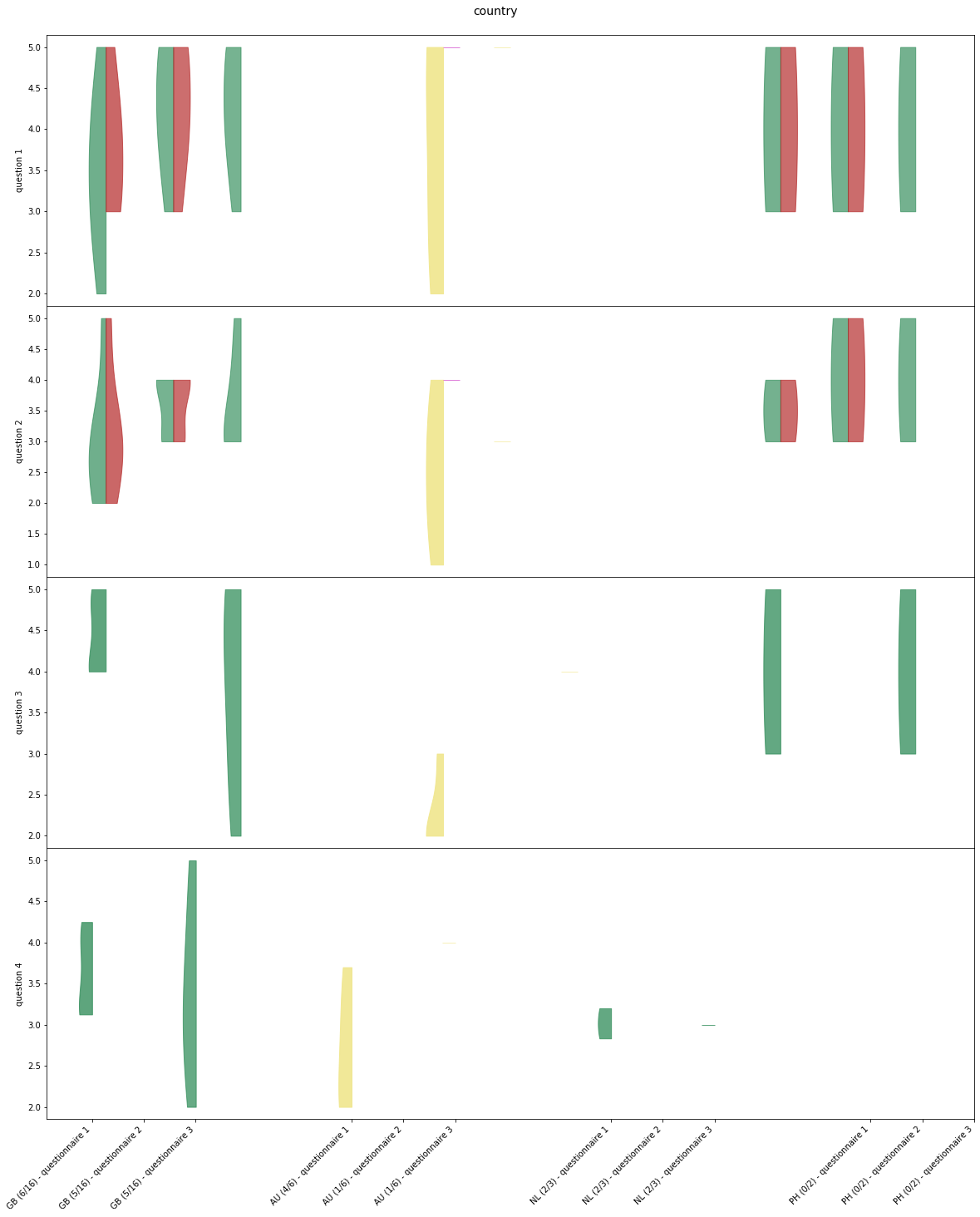Figure 8: Violin Graphs for Country Groupings. We can see a more varied number of trends, some trends going up and some going down. Yet, with the current data, the statistical significance can be often lacking.

### Generalized Statistics for Steps¶

Now that we have built a generalized statistics function call for plotting participant table column groupings against participant responses we can do the same against started MOOC steps. We start with the general graph.

In :
ticks = list(range(len(all_step)))
labels = list(map(lambda x: f'week {x+1}', ticks)) + ['total']
ticks += [len(all_step)]
colors_left = ['khaki'] * len(all_step) + ['seagreen']
colors_right = ['orchid'] * len(all_step) + ['firebrick']
all_group = all_step + [all_step.append(all_step[1:])]
fil = lambda x: x.groupby('participant_id').sum().started
left = list(map(fil, all_group))
atmp = np.array(list(map(len, left)))
alpha = atmp / (atmp.sum() * 2) + .5  # weight size for alpha values

fig, ax = plt.subplots(figsize=(20, 12))
double_violin(left, right, ticks, ax=ax,
color_left=colors_left, color_right=colors_right, alpha=alpha)
ax.set_xticks(ticks)
ax.set_xticklabels(labels, rotation=45, ha='right')
ax.set_ylabel('number of participants who started n steps')
ax.set_title('Steps Started');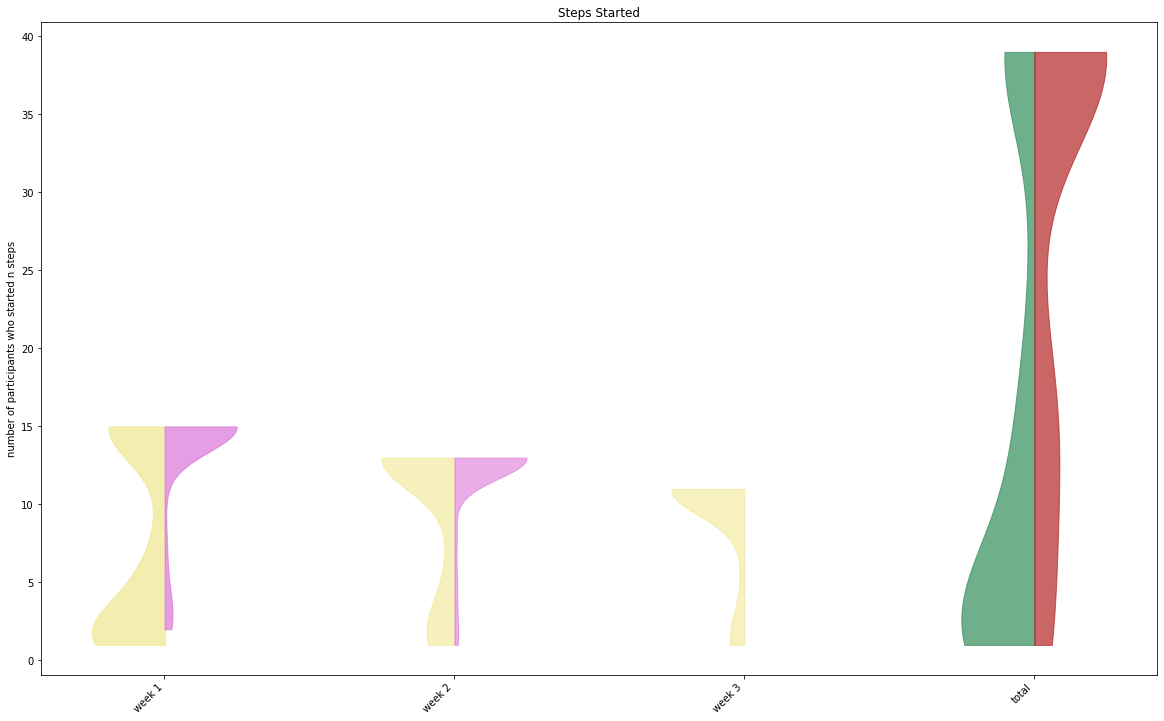Figure 9: Number of Total Started Steps across Weeks. Here we see two well known trends, first, participants either give up early completing very few steps or complete the majority of the steps for the week. Second, we see that the majority of participants give up after a few steps in the first week. Note that we have a graph for every week and then one graph for the grand total of steps. The half-violins are read in the same way as with questionnaires: the left half-violin is for all participants whilst the right one is for the participants that advanced for the following week.

A new trend visible in this specific data is the set of participants that did start the same step several times, many of these participants did not advance for the next week. We can see this trend in the grand total double-violin where the left-half violin is higher. The trend suggests that many participants may prefer to mix and match the MOOC steps at their own pace rather than following the steps in order.

Next we build the generalized statistics graph function call as we do for responses. For the steps we have week graphs and a grand total graph, hence we will plot a set of violin graphs for a single grouping in a vertical fashion. This is different from the graph just above.

In :
# to keep things readable, we limit by 13 biggest values by default
def plot_step(stat, start=0, limit=13):
total = all_step.append(all_step[1:])
n_weeks = total.week.max()
estat = participant[stat].value_counts()
fig, ax = plt.subplots(n_weeks + 1, 1, sharex=True, figsize=(20, 6*n_weeks + 6))

colors_left = (['khaki', 'seagreen'] * len(estat))[:len(estat)]
colors_right = (['orchid', 'firebrick'] * len(estat))[:len(estat)]
ticks = list(range(len(estat)))
if limit:
ticks = ticks[start:limit]
ylabels = list(map(lambda x: f'week {x+1}', range(n_weeks))) + ['total']
complete_ticks = []
labels = estat.index
if limit:
labels = labels[start:limit]
all_group = all_step + [all_step.append(all_step[1:])]
for i in range(n_weeks + 1):
walk = enumerate(estat.index)
if limit:
walk = list(walk)[start:limit]
left = []
right = []
for n_stat, cur_stat in walk:
fil = lambda x: x[x[stat] == cur_stat].groupby('participant_id').sum().started
left.append(fil(all_group[i]))
# weight size for alpha values (prevent division by zero)
atmp = np.array(list(map(len, left)))
alpha = atmp / (atmp.sum() * 2 + .1) + .5
double_violin(left, right, ticks, ax=ax[i],
color_left=colors_left, color_right=colors_right, alpha=alpha)
ax[i].set_ylabel(ylabels[i])

ax[-1].set_xticks(ticks);
ax[-1].set_xticklabels(labels, rotation=45, ha='right')
fig.suptitle(f'Steps started by {stat}', y=.9, fontsize=14)
return estat


The call plot_step is analogous and has the same arguments and usage as plot_response. This includes the start= and limit= optional arguments.

For example, using the groupings for education levels in the current data.

In :
plot_step('highest_education_level')

Out:
Unknown                 330
university_degree        28
university_masters       15
tertiary                  6
university_doctorate      4
secondary                 3
Name: highest_education_level, dtype: int64